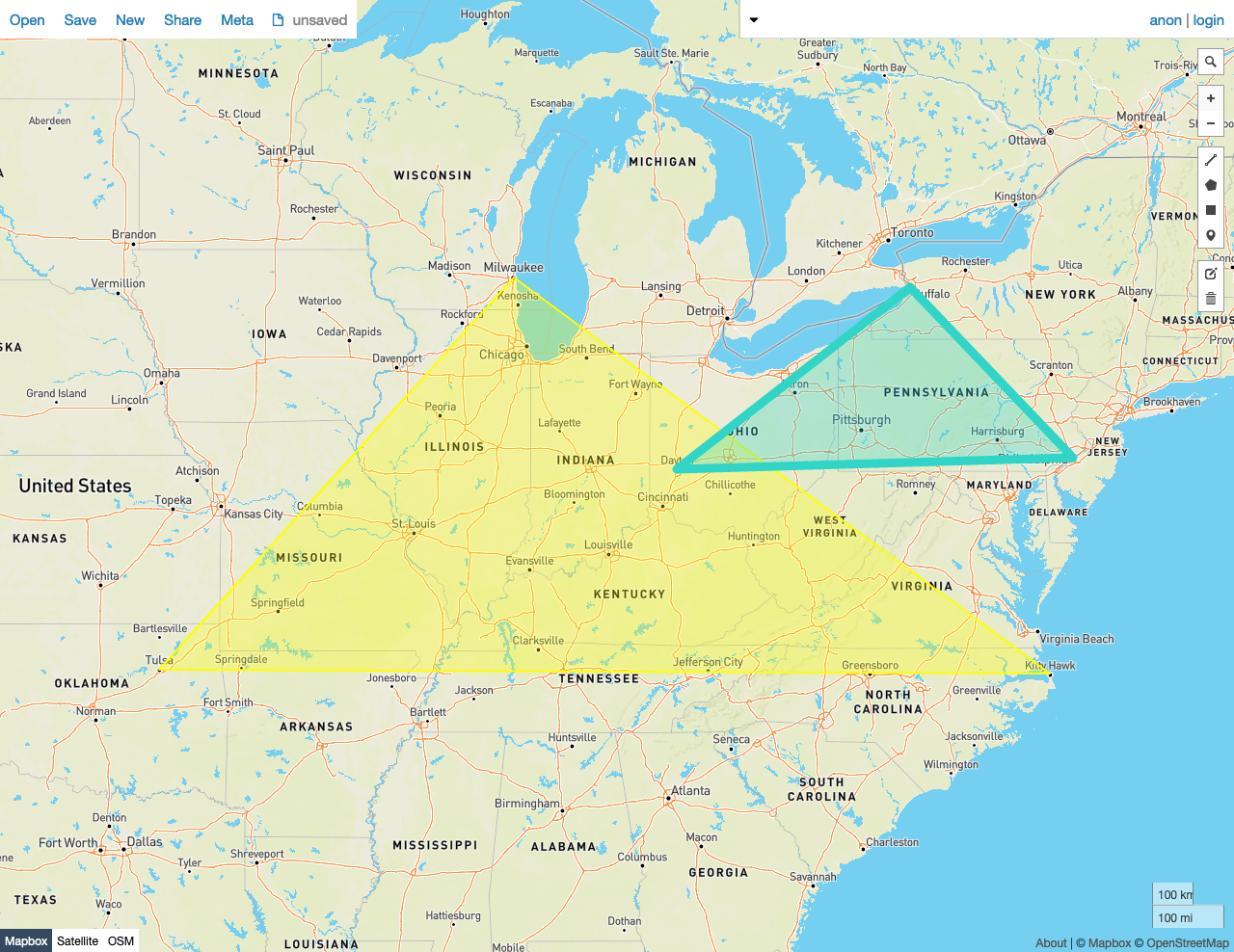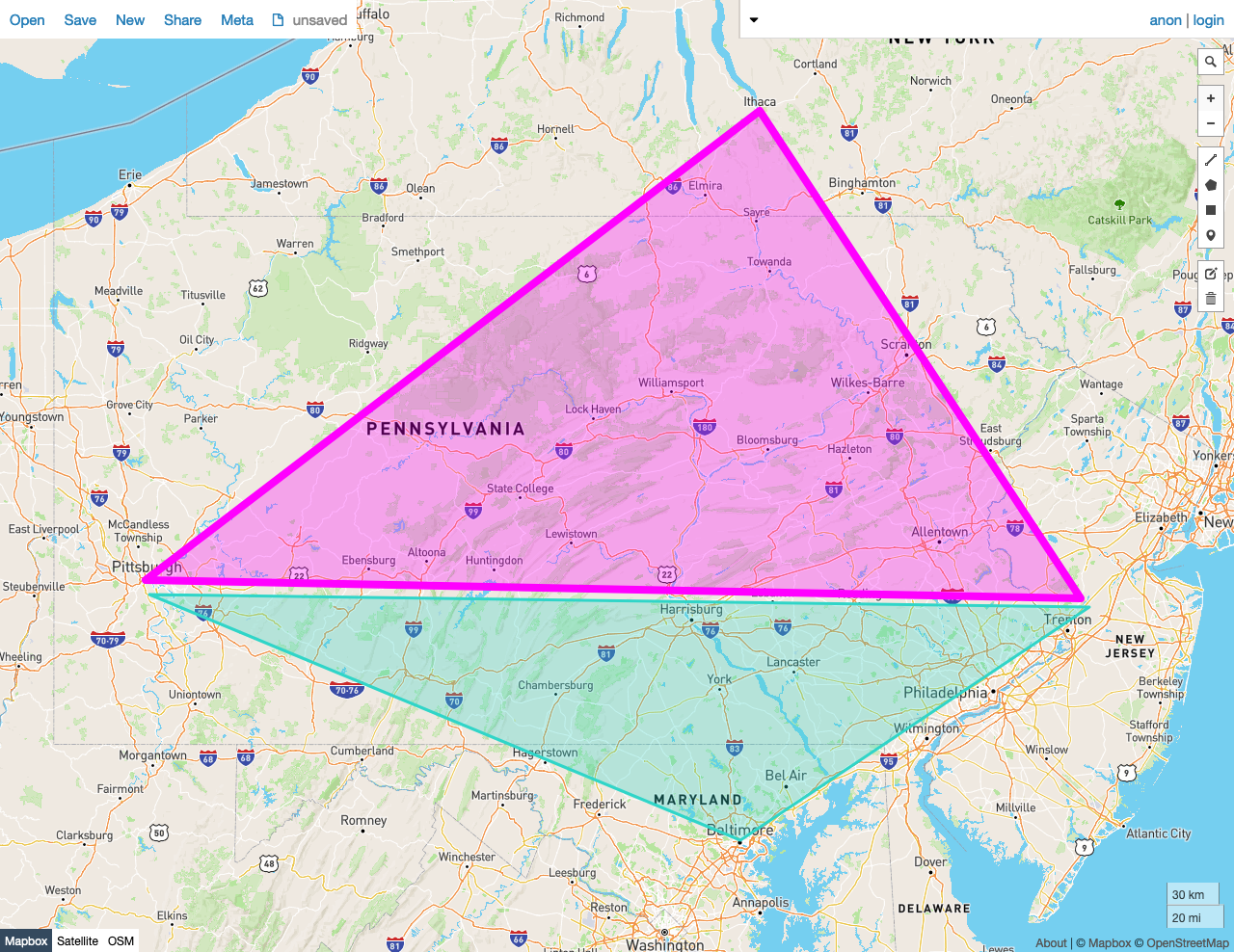Given two shapes A and B, ST_Overlaps(A, B) returns true if the following criteria are met:

• The shapes share any of the same space -- that is, if any point in the set that comprises A is also a member of the set of points that make up B.
• Neither of the shapes is contained by the other, in the ST_Contains sense.

In other words, ST_Overlaps returns true if the shapes intersect (in the ST_Intersects sense), but neither is contained within the other.

ST_Overlaps works on the following spatial data types:

Note:

ST_Overlaps will attempt to use any available spatial index to speed up its operation. Use the prefixed variant _ST_Overlaps if you do not want any spatial indexes to be used.

Examples

Note:

The screenshots in these examples were generated using geojson.io, but they are designed to showcase the shapes, not the map. Representing GEOMETRY data in GeoJSON can lead to unexpected results if using geometries with SRIDs other than 4326 (as shown below).

True

In this example, ST_Overlaps returns true because:

• There is a Point in the set that comprises Polygon A that is also a member of the set of Points that make up Polygon B.
• Neither of the shapes is contained by the other, in the ST_Contains sense.
SELECT st_overlaps(st_geomfromtext('SRID=4326;POLYGON((-87.906471 43.038902, -95.992775 36.153980, -75.704722 36.076944, -87.906471 43.038902))'), st_geomfromtext('SRID=4326;POLYGON((-84.191605 39.758949, -75.165222 39.952583, -78.878738 42.880230, -84.191605 39.758949))'));
st_overlaps
---------------
true

(1 row)False

In this example, ST_Overlaps returns false because:

• - There is not any Point in the set that comprises Polygon A that is also a member of the set of Points that make up Polygon B.
SELECT st_overlaps(st_geomfromtext('SRID=4326;POLYGON((-79.995888 40.440624,-74.666728 40.358244, -76.5 42.443333, -79.995888 40.440624))'), st_geomfromtext('SRID=4326;POLYGON((-79.976111 40.374444, -74.621157 40.323294, -76.609383 39.299236, -79.976111 40.374444))'));
st_overlaps
---------------
false
(1 row)